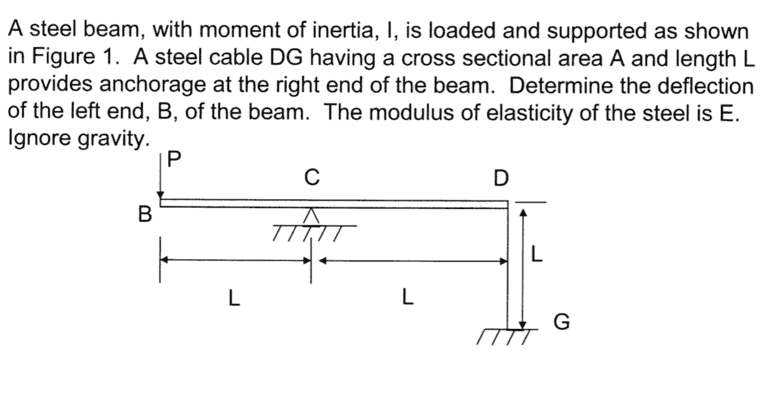# Calculating deflection with rotation about center support

I am preparing for a qualifying exam for my PhD program and am looking at some of the old tests from previous years (as supplied by the school for study/prep material). I have come across a deflection problem that has me stumped, and I might just be overthinking it.

The problem:SteamKing
Staff Emeritus
Homework Helper
I am preparing for a qualifying exam for my PhD program and am looking at some of the old tests from previous years (as supplied by the school for study/prep material). I have come across a deflection problem that has me stumped, and I might just be overthinking it.

The problem:
View attachment 98034
The first thing to do is draw a free body diagram of the beam.

You may be overthinking this problem indeed, but you haven't shown any work.

The first thing to do is draw a free body diagram of the beam.

You may be overthinking this problem indeed, but you haven't shown any work.

Sorry. I should have elaborated more on where I was stuck.

I did the free body diagram, summed the forces and then summed the moments at C and found the force due to the cable was equal to the applied force, and the reaction force at the support was 2P. The second free body diagram of the cable shows the force in the cable is P and in tension. (And the reaction force at G is also P)

I know that the deflection at D in the cable has to be equal to the deflection in the beam at D.

So where I'm stuck.
Typically Deflection in a cable can be simplified to (PL)/(Acable*E).

For a beam I usually use the Castigliano Theorem to find the deflection based on the strain energy in the beam.

So I guess I'm just unsure of how to proceed from here. Do I do just Castigliano and do the integral of the moment across the beam and add the integral of the axial force in the cable along the cable (which would vein the opposite direction of the deflection of the beam at that point)? Or do I do Castigliano of the beam and set it equal to the deflection of the cable? And how do I equate for rotation?

Or do I do Castigliano from C to D, multiply by 2 to get the point at B due to symmetry and rotation, then add the deflection of the cable?

When I think of total strain energy of the system I think of a set ammount of energy going into the system and some going into the cable and some going into the beam. This would imply that it's not adding all the deflections. Or is it that with more material in the beam plus the cable there is the capacitance to absorb MORE energy so the total energy is increased by the cable?

Ive been studying non-stop for a week for the upcoming exams and looking at a plethora of different topics, so my mind is mush now. I'm sure this is simple and I've gone WAY over board in trying to cenceptualize and think through the theory. I just thought around so many loops on this that I got lost.

First, calculate the reactions, then I'd suggest using the singularity functions to calculate the deflection at point B (remember that you can turn around the beam to keep the cable on the right). Then you'd have 2 boundary conditions y(L)=0 and Y(0)= deformation of the cable due to the reaction force.

On the other thand you could use the finite element method using 2 beam elements to model the beam and a spring to model the cable.